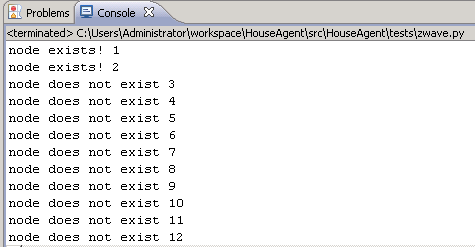## Z-wave and python node discovery, more bitwise trickery

This post continues upon the last blog post, in the last post we looked at some z-wave protocol basics.
At the same time we had a look at a bitwise operation (XOR), in this post some more bitwise tricks will be used to do z-wave node discovery.
Node discovery is essential to get information about the members of a z-wave network.
The serial port sniff in the previous post, showed the FUNC_ID_SERIAL_API_GET_INIT_DATA command. Let’s dive into this packet…
Here’s the packet response again:
#06#01#25#01#02#05#00#1D#03#00#00#00#00#00#00#00#00#
00#00#00#00#00#00#00#00#00#00#00#00#00#00#00#00#00#00#00#00#03#01#C0

The first 4 bytes are not relevant for this post, I have to look into them later on. The fun starts at byte “02”, this byte represents the function response code (in this case FUNC_ID_SERIAL_API_GET_INIT_DATA). The byte following (05) is the z-wave protocol version, in this case v5. Next byte is “00” which is the controller type (primary or secondary)
“1D” indicates the amount of node bytes. 1D is 29 decimal.
Z-wave uses these node bytes to indicate whether or not a node is in use within the network (the bytes are bit masked). For example the first node byte value 03, is as follows in binary:
0000 0011

This means node 1 and 2 are in use, another example:

0000 1011

This would indicate node 4, 2 and 1 being used. So, how do we figure this out from code? This is where bitwise AND comes into play. A bitwise AND takes two binary representations of equal length and performs the logical AND operation on each pair of corresponding bits. In each pair, the result is 1 if the first bit is 1 AND the second bit is 1. Otherwise, the result is 0.
We can use this from our python code in the following matter:

```    length = ord(response) - 1
index = 1

for i in range(0, length):
buffer_index = response[8+i]
num = 1
j = 0

while j <= 7:
if (ord(buffer_index) & num > 0):
print "node exists!", index
else:
print "node does not exist", index

index = index+1

if j < 7:
num = num * 2

j = j+1
```

And does it work?Looks fine!

You can leave a response, or trackback from your own site.

### 2 Responses to “Z-wave and python node discovery, more bitwise trickery”

1.LDS says:

Hi!

I’m very interested in your investigation regarding the Z-Wave protocol.

I would like to ask you a few questions if possible.

You state that: “The first 4 bytes are not relevant for this post, I have to look into them later on. The fun starts at byte “02″, this byte represents the function response code (in this case FUNC_ID_SERIAL_API_GET_INIT_DATA). The byte following (05) is the z-wave protocol version, in this case v5. Next byte is “00″ which is the controller type (primary or secondary)
“1D” indicates the amount of node bytes. 1D is 29 decimal.”

My questions are: how do you know what byte “02” means? and how do you know its name? The same questions for byte “05”, “00” and “1D”.

I appreciate the attention, thank you! :D

Cheers,
LDS

2.melchoir55 says:

Has any further work been done here? I am keenly interested in interpreting the data being sent from a few zwave devices. I’d appreciate references to any documentation you have created or know about.